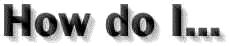###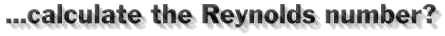The Reynolds number is an important value for the behavior of the flow, and especially the boundary layer. Flows with the same Reynolds number behave similar. This number can be calculated by the exact formula, given in the glossary. If medium accuracy is sufficient, the Reynolds number can be approximately calculated by the following formula.

 Re = v * l * 70000
where:
 v flight speed [m/s] l chord length [m] 70000 constant value (for air) [s/m²]

The Reynolds number is based on a length, which is usually the chord length of an airfoil (in two dimensions) or the chord length of a wing. Because the chord length of a wing may vary from root to tip, a mean aerodynamic chord length is used to define the Reynolds number for a wing. Reynolds numbers are also used for other objects, like the flows in tubes, where other length scales are used. For the flow in tubes or around a sphere, the diameter is used.

Example: A full scale aircraft flies at a speed of 100 m/s and has a tip chord of 1 meter an a root chord of 2.5 meters. The Reynolds number of the flow at the tip station is approximately 100 * 1.0 * 70000 = 7 Millions, at the root the Reynolds number is 17.5 Millions. A 1/4 scale model would fly at roughly 40 m/s and thus would have a tip Reynolds number of 40 * 1.0/4 * 70000 = 0.7 Millions, which is only 1/10 of the full scale plane. Because of this difference, we have to expect a different flow behavior in terms of drag and stall characteristics.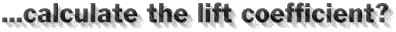The lift coefficient is a relative measure for the lift of an airfoil or wing. It is used for comparisons of wings of different size and at different conditions (speed, density of air). Similar coefficients are also used for drag and moment. Its definition reads: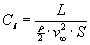where:
 L lift [N] v flight speed [m/s] S wing area [m²]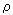density of air [kg/m³]

Example: We want to build a heavy airplane model which will have an assumed mass of 10.0 kg. Since it is a scale model, its wing area is already defined to be 0.8 m². From our experience with similar models, we have chosen an airfoil, and want to know, how slow we can come in for landing. The wing has to lift the weight of the model (mass*9.81) and we hope to flare out at a speed of 10 m/s. Thus, the necessary lift coefficient would be 10.0*9.81/(1.225/2 * 10*10 * 0.8) = 2.0. If we look into the polar charts for the selected airfoil, we find, that its maximum lift coefficient is about 1.5 and thus lower, than the required value of 2.0. This means, we cannot fly as slow as we wanted. If we could reduce the weight to 7.5 kg, the required lift coefficient would go down to 1.5, which is what we can achieve with the selected airfoil.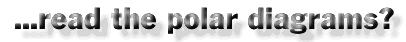Due to the increasing amount of SPAM mail, I have to change this e-Mail address regularly. You will always find the latest version in the footer of all my pages.

It might take some time until you receive an answer and in some cases you may even receive no answer at all. I apologize for this, but my spare time is limited. If you have not lost patience, you might want to send me a copy of your e-mail after a month or so.
This is a privately owned, non-profit page of purely educational purpose. Any statements may be incorrect and unsuitable for practical usage. I cannot take any responsibility for actions you perform based on data, assumptions, calculations etc. taken from this web page.